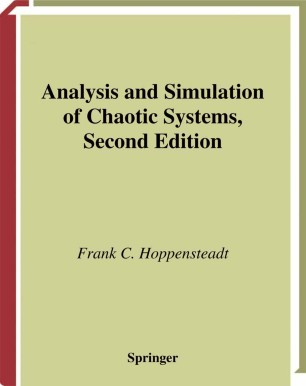# Analysis and Simulation of Chaotic SystemsTextbook

Part of the Applied Mathematical Sciences book series (AMS, volume 94)

1. Front Matter
Pages i-xx
2. Pages 1-25
3. Pages 27-89
4. Pages 91-120
5. Pages 121-143
6. Pages 145-161
7. Pages 163-194
8. Pages 195-247
9. Pages 249-300
10. Back Matter
Pages 301-317

### Introduction

Beginning with realistic mathematical or verbal models of physical or biological phenomena, the author derives tractable mathematical models that are amenable to further mathematical analysis or to elucidating computer simulations. For the most part, derivations are based on perturbation methods. Because of this, the majority of the text is devoted to careful derivations of implicit function theorems, the method of averaging, and quasi-static state approximation methods. The duality between stability and perturbation is developed and used, relying heavily on the concept of stability under persistent disturbances. This explains why stability results developed for quite simple problems are often useful for more complicated, even chaotic, ones. Relevant topics about linear systems, nonlinear oscillations, and stability methods for difference, differential-delay, integro- differential and ordinary and partial differential equations are developed throughout the book. For the second edition, the author has restructured the chapters, placing special emphasis on introductory materials in Chapters 1 and 2 as distinct from presentation materials in Chapters 3 through 8. In addition, more material on bifurcations from the point of view of canonical models, sections on randomly perturbed systems, and several new computer simulations have been added.

### Keywords

Chaotic Systems Implicit function Mathematical Models Nonlinear system bifurcation calculus computer differential equation simulation